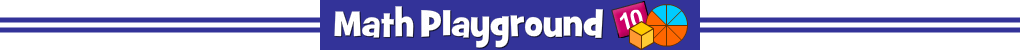Ratio Martian
What exactly is a ratio? Would you recognize one if it wandered past you?
Practice identifying ratios in this sci-fi math game!
Ratio Martian - Learning Connections
Essential Skills
Mental Math - identify ratios
Problem Solving - assess various math expressions

Common Core Connection for Grades 6 and 7
Understand ratio concepts and use ratio reasoning to solve problems.
More Math Games to Play